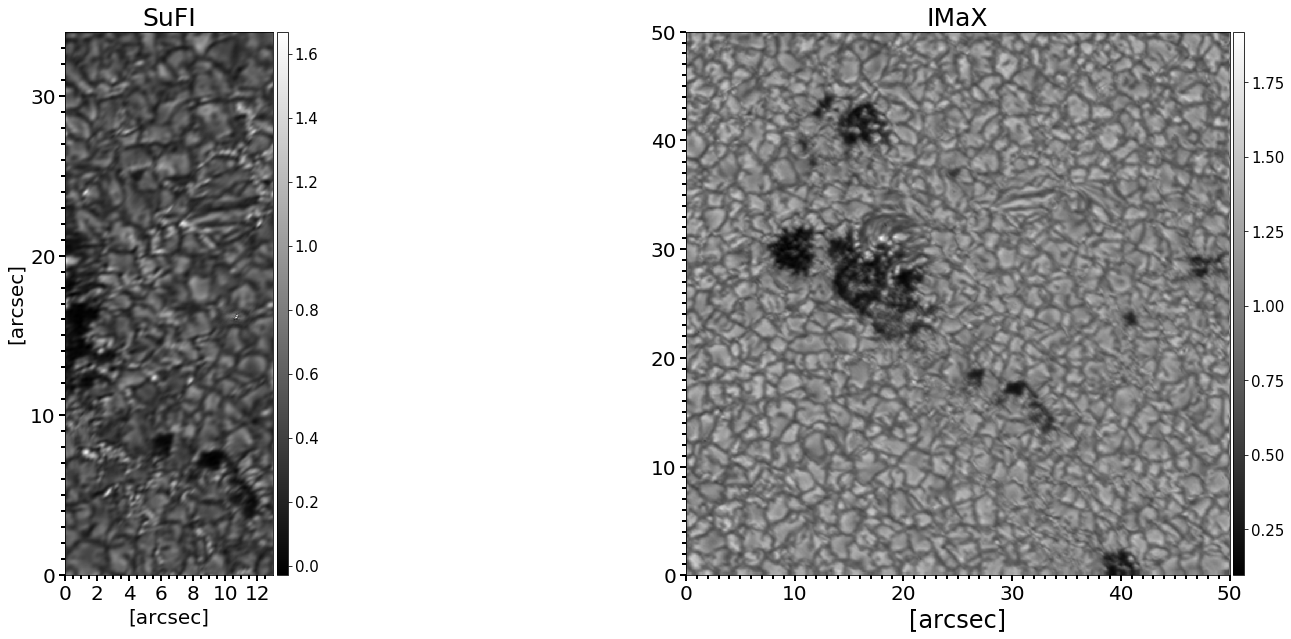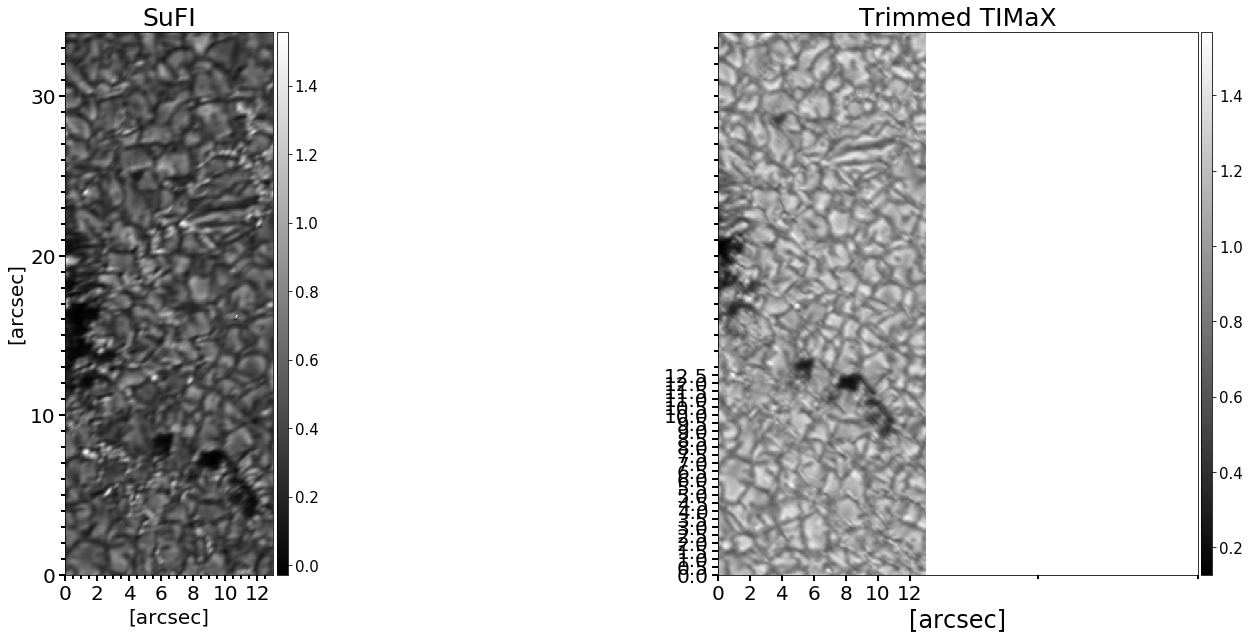# Image registration

## Image Alignment¶

#### In this tutorial, I will show an example of aligning two data sets, each with different pixel size and field of view dimensions.¶

In :
## importing libraries
import pyfits
import numpy as np
import matplotlib.pyplot as plt
import pylab
from mpl_toolkits.axes_grid1 import make_axes_locatable, axes_size
from numpy import flipud


#### For this example, I have a brightness image taken by an instrument called SuFI, which is a filtergraph on-board the balloon observatory, SUNRISE. The other continuum image is from another scientific instrument called IMaX, which is a spectropolarimter. SuFI FOV is smaller and a sub of the IMaX FOV as you can see below.¶

In :
path = '/home/fatima/Desktop/project_2/alignment/'
sufi_image = np.flipud(pyfits.getdata(path+'sufi_007.fits'))
imax_image = np.flipud(pyfits.getdata(path+'imax_007.fits')) ## we are flipping the images for a better view

/usr/local/lib/python2.7/dist-packages/pyfits/card.py:704: UserWarning: The following header keyword is invalid or follows an unrecognized non-standard convention:
REDPDVER =                   '1.1' / Phase Diversity reduction version
self._image)
/usr/local/lib/python2.7/dist-packages/pyfits/card.py:704: UserWarning: The following header keyword is invalid or follows an unrecognized non-standard convention:
L_BURST  =                       1 / Number of images of burst
self._image)
/usr/local/lib/python2.7/dist-packages/pyfits/card.py:704: UserWarning: The following header keyword is invalid or follows an unrecognized non-standard convention:
BURST    =  / Image Numbers of burst
self._image)
/usr/local/lib/python2.7/dist-packages/pyfits/card.py:704: UserWarning: The following header keyword is invalid or follows an unrecognized non-standard convention:
AVZER    =                       1 /  use averaged Zernikes: yes=1, no=0
self._image)
/usr/local/lib/python2.7/dist-packages/pyfits/card.py:704: UserWarning: The following header keyword is invalid or follows an unrecognized non-standard convention:
AVZERFIL = 'avZernike_0000839-0001352.sav' /  filename containing averaged Zerni
self._image)
/usr/local/lib/python2.7/dist-packages/pyfits/card.py:704: UserWarning: The following header keyword is invalid or follows an unrecognized non-standard convention:
TELEDIAM =                   1.000 / (m) Telescope diameter
self._image)
/usr/local/lib/python2.7/dist-packages/pyfits/card.py:704: UserWarning: The following header keyword is invalid or follows an unrecognized non-standard convention:
FOCALLEN =                   121.6 / (m) Telescope focal length
self._image)
/usr/local/lib/python2.7/dist-packages/pyfits/card.py:704: UserWarning: The following header keyword is invalid or follows an unrecognized non-standard convention:
DEFOCUS  =                   28.15 / (mm) Defocus distance
self._image)
/usr/local/lib/python2.7/dist-packages/pyfits/card.py:704: UserWarning: The following header keyword is invalid or follows an unrecognized non-standard convention:
NRZERNIK =                      45 / Max. Zernike coefficient
self._image)
/usr/local/lib/python2.7/dist-packages/pyfits/card.py:704: UserWarning: The following header keyword is invalid or follows an unrecognized non-standard convention:
SCALEFAC =                  1.0208 / ratio of platescales foc/defoc
self._image)
/usr/local/lib/python2.7/dist-packages/pyfits/card.py:704: UserWarning: The following header keyword is invalid or follows an unrecognized non-standard convention:
INT_SUB  =                       1 / subpixel shift foc/defoc: yes=1, no=0
self._image)
/usr/local/lib/python2.7/dist-packages/pyfits/card.py:704: UserWarning: The following header keyword is invalid or follows an unrecognized non-standard convention:
X_FOC    =                       0 / (pxl) x-position of focussed image
self._image)
/usr/local/lib/python2.7/dist-packages/pyfits/card.py:704: UserWarning: The following header keyword is invalid or follows an unrecognized non-standard convention:
Y_FOC    =                       0 / (pxl) y-position of focussed image
self._image)
/usr/local/lib/python2.7/dist-packages/pyfits/card.py:704: UserWarning: The following header keyword is invalid or follows an unrecognized non-standard convention:
X_DEFOC  =                     965 / (pxl) x-position of defocussed image
self._image)
/usr/local/lib/python2.7/dist-packages/pyfits/card.py:704: UserWarning: The following header keyword is invalid or follows an unrecognized non-standard convention:
Y_DEFOC  =                       0 / (pxl) y-position of defocussed image
self._image)
/usr/local/lib/python2.7/dist-packages/pyfits/card.py:704: UserWarning: The following header keyword is invalid or follows an unrecognized non-standard convention:
N_OVERLX =                       1 / add. subboxes defining overlapp x
self._image)
/usr/local/lib/python2.7/dist-packages/pyfits/card.py:704: UserWarning: The following header keyword is invalid or follows an unrecognized non-standard convention:
N_OVERLY =                       1 / add. subboxes defining overlapp y
self._image)
/usr/local/lib/python2.7/dist-packages/pyfits/card.py:704: UserWarning: The following header keyword is invalid or follows an unrecognized non-standard convention:
X_BOXES  = [0,1,2,3,4,5,6] / reconstructed subboxes x
self._image)
/usr/local/lib/python2.7/dist-packages/pyfits/card.py:704: UserWarning: The following header keyword is invalid or follows an unrecognized non-standard convention:
Y_BOXES  = [0,1,2,3,4,5,6,7,8,9,10,11,12,13,14,15] / reconstructed subboxes y
self._image)
/usr/local/lib/python2.7/dist-packages/pyfits/card.py:704: UserWarning: The following header keyword is invalid or follows an unrecognized non-standard convention:
SIZESUB  =                     256 / (pxl) Size of subimages
self._image)
/usr/local/lib/python2.7/dist-packages/pyfits/card.py:704: UserWarning: The following header keyword is invalid or follows an unrecognized non-standard convention:
APODIZ   =                   25.00 / (%) width of apodization rim
self._image)
/usr/local/lib/python2.7/dist-packages/pyfits/card.py:704: UserWarning: The following header keyword is invalid or follows an unrecognized non-standard convention:
CON_TRAN =                      11 / (pxl) transition region while connecting
self._image)
/usr/local/lib/python2.7/dist-packages/pyfits/card.py:704: UserWarning: The following header keyword is invalid or follows an unrecognized non-standard convention:
CCDMTF   =                       1 / MTF of CCD: yes=1, no=0
self._image)
/usr/local/lib/python2.7/dist-packages/pyfits/card.py:704: UserWarning: The following header keyword is invalid or follows an unrecognized non-standard convention:
COBS     =                   0.332 / Central Obscuration (mask=-1)
self._image)
/usr/local/lib/python2.7/dist-packages/pyfits/card.py:704: UserWarning: The following header keyword is invalid or follows an unrecognized non-standard convention:
FILCLASS =                       0 / Noise filter class
self._image)
/usr/local/lib/python2.7/dist-packages/pyfits/card.py:704: UserWarning: The following header keyword is invalid or follows an unrecognized non-standard convention:
CUTOFF   =                   0.100 / (arcsec) Noise filter cut-off (class 3)
self._image)
/usr/local/lib/python2.7/dist-packages/pyfits/card.py:704: UserWarning: The following header keyword is invalid or follows an unrecognized non-standard convention:
FILTFACT =                   1.000 / Noise filterfactor
self._image)
/usr/local/lib/python2.7/dist-packages/pyfits/card.py:704: UserWarning: The following header keyword is invalid or follows an unrecognized non-standard convention:
LOW_F    =                   0.200 / Noise filter bottom (cf thesis Loefdahl)
self._image)
/usr/local/lib/python2.7/dist-packages/pyfits/card.py:704: UserWarning: The following header keyword is invalid or follows an unrecognized non-standard convention:
REGEPS   =                   0.050 / Additive contrib. to Q
self._image)
/usr/local/lib/python2.7/dist-packages/pyfits/card.py:704: UserWarning: The following header keyword is invalid or follows an unrecognized non-standard convention:
D_PIX    =                   1.000 / (pxl) reduction of cut_off frequency
self._image)
/usr/local/lib/python2.7/dist-packages/pyfits/card.py:704: UserWarning: The following header keyword is invalid or follows an unrecognized non-standard convention:
W_CUT    =                   0.010 / SVD ( 0. < w_cut < 1. )
self._image)
/usr/local/lib/python2.7/dist-packages/pyfits/card.py:704: UserWarning: The following header keyword is invalid or follows an unrecognized non-standard convention:
RMS_LIM  =                   0.800 / SVD rms limit
self._image)
/usr/local/lib/python2.7/dist-packages/pyfits/card.py:704: UserWarning: The following header keyword is invalid or follows an unrecognized non-standard convention:
NSING    =                   6.000 / SVD singularities limit
self._image)
/usr/local/lib/python2.7/dist-packages/pyfits/card.py:704: UserWarning: The following header keyword is invalid or follows an unrecognized non-standard convention:
ITERAMIN =                       3 / Min. number of iterations
self._image)
/usr/local/lib/python2.7/dist-packages/pyfits/card.py:704: UserWarning: The following header keyword is invalid or follows an unrecognized non-standard convention:
ITERAMAX =                     150 / Max. number of iterations
self._image)
/usr/local/lib/python2.7/dist-packages/pyfits/card.py:704: UserWarning: The following header keyword is invalid or follows an unrecognized non-standard convention:
PHIEPS   =                  0.0100 / Convergence limit of delta_phi_rms
self._image)
/usr/local/lib/python2.7/dist-packages/pyfits/card.py:704: UserWarning: The following header keyword is invalid or follows an unrecognized non-standard convention:
LEPS     =                  0.0000 / Convergence limit of abs(delta(L_M)/L_M)
self._image)
/usr/local/lib/python2.7/dist-packages/pyfits/card.py:704: UserWarning: The following header keyword is invalid or follows an unrecognized non-standard convention:
X_OFFSET =                      64 / x lower left wrt. raw data (pxl)
self._image)
/usr/local/lib/python2.7/dist-packages/pyfits/card.py:704: UserWarning: The following header keyword is invalid or follows an unrecognized non-standard convention:
Y_OFFSET =                      64 / y lower left wrt. raw data (pxl)
self._image)

In :
fig=plt.figure(figsize=(25,10),facecolor='white')
aspect = 5

im=ax.imshow(sufi_image, extent=[0,13,0,34],cmap=pylab.gray())
ax.set_xticks(np.arange(0,13,2))
ax.set_xticks(np.arange(0,13,0.5),minor=True)
ax.set_yticks(np.arange(0,34,10))
ax.set_yticks(np.arange(0,34,1),minor=True)
ax.tick_params(axis = 'both', which = 'major',length=6, width=2,labelsize = 20)
ax.tick_params(axis = 'both', which = 'minor', length=4, width=2)
ax.set_xlabel('[arcsec]',fontsize=20)
ax.set_ylabel('[arcsec]',fontsize=20)
ax.set_title('SuFI',fontsize=25)
divider = make_axes_locatable(ax)
cbar = plt.colorbar(im, cax=cax,orientation='vertical')#,ticks=np.arange(0.4,0.9,0.1))
cax.tick_params(labelsize=15)

im2=ax2.imshow(imax_image, extent=[0,50,0,50],cmap=pylab.gray())
ax2.set_title('IMaX')
ax2.set_xticks(np.arange(0,51,10))
ax2.set_yticks(np.arange(0,51,10))
ax2.set_xticks(np.arange(0,51,1),minor=True)
ax2.set_yticks(np.arange(0,51,1),minor=True)
ax2.tick_params(axis = 'both', which = 'major',length=6, width=2,labelsize = 20)
ax2.tick_params(axis = 'both', which = 'minor', length=4, width=2)
ax2.set_title('IMaX',fontsize=25)
ax2.set_xlabel('[arcsec]',fontsize=24)
#ax.set_ylabel('[arcsec]',fontsize=20)
divider2 = make_axes_locatable(ax2)
cbar2 = plt.colorbar(im2, cax=cax2,orientation='vertical')#,ticks=np.arange(0.4,0.9,0.1))
cax2.tick_params(labelsize=15)

plt.show()

## print the dimensions of the images
print sufi_image.shape
print imax_image.shape(1913, 776)
(936, 936)


#### For pixel size resampling, I will use the code Resampling where we used bi-linear interpolation to change the pixel size.¶

In :
from scipy import interpolate

def Resample(data1,pixel_size_1,pixel_size_2):

len_X = len(data1[1,:])
len_Y = len(data1[:,1])
p = pixel_size_2/pixel_size_1
xnew = np.arange(0, len_X, p)
ynew = np.arange(0,len_Y,p)
f= interpolate.interp2d(np.arange(len_X), np.arange(len_Y),data1, kind='linear')
resamp_data1 = f(xnew,ynew)
return resamp_data1, resamp_data1.shape

In :
sufi_resamp, sufi_resamp_shape = Resample(sufi_image, 0.02, 0.05)
print sufi_resamp_shape ## size of the resampled SuFI image

(766, 311)


#### As a second step I will be manually trimming IMaX image to match the size and features of SuFI.¶

In :
imax_trim = imax_image[120:886,370:681]

In :
fig=plt.figure(figsize=(25,10),facecolor='white')
aspect = 5

im=ax.imshow(sufi_resamp, extent=[0,13,0,34],cmap=pylab.gray())
ax.set_xticks(np.arange(0,13,2))
ax.set_xticks(np.arange(0,13,0.5),minor=True)
ax.set_yticks(np.arange(0,34,10))
ax.set_yticks(np.arange(0,34,1),minor=True)
ax.tick_params(axis = 'both', which = 'major',length=6, width=2,labelsize = 20)
ax.tick_params(axis = 'both', which = 'minor', length=4, width=2)
ax.set_xlabel('[arcsec]',fontsize=20)
ax.set_ylabel('[arcsec]',fontsize=20)
ax.set_title('SuFI',fontsize=25)
divider = make_axes_locatable(ax)
cbar = plt.colorbar(im, cax=cax,orientation='vertical')#,ticks=np.arange(0.4,0.9,0.1))
cax.tick_params(labelsize=15)

im2=ax2.imshow(imax_trim, extent=[0,13,0,34],cmap=pylab.gray())
ax2.set_xticks(np.arange(0,13,2))
ax2.set_yticks(np.arange(0,13,0.5))
ax2.set_xticks(np.arange(0,34,10),minor=True)
ax2.set_yticks(np.arange(0,34,1),minor=True)
ax2.tick_params(axis = 'both', which = 'major',length=6, width=2,labelsize = 20)
ax2.tick_params(axis = 'both', which = 'minor', length=4, width=2)
ax2.set_title('Trimmed TIMaX',fontsize=25)
ax2.set_xlabel('[arcsec]',fontsize=24)
#ax.set_ylabel('[arcsec]',fontsize=20)
divider2 = make_axes_locatable(ax2)
cbar2 = plt.colorbar(im2, cax=cax2,orientation='vertical')#,ticks=np.arange(0.4,0.9,0.1))
cax2.tick_params(labelsize=15)

plt.show()#### Now that the two images have the same dimensions, we carry on with the image alignment . We will try to align the trimmed IMaX to the resampled SufI image. For this we will be using the package imreg_dft¶

In :
import imreg_dft


#### This module is not installed, we install it by typing:¶

In :
! pip install --user imreg_dft

Requirement already satisfied: imreg_dft in /home/fatima/.local/lib/python2.7/site-packages (2.0.0)
Requirement already satisfied: scipy>=0.12 in /usr/local/lib/python2.7/dist-packages (from imreg_dft) (0.19.0)
Requirement already satisfied: pillow in /usr/local/lib/python2.7/dist-packages (from imreg_dft) (3.4.2)
Requirement already satisfied: setuptools>=1.0 in /usr/local/lib/python2.7/dist-packages (from imreg_dft) (36.0.1)
Requirement already satisfied: numpy>=1.7 in /usr/local/lib/python2.7/dist-packages (from imreg_dft) (1.13.0)
You are using pip version 18.0, however version 19.1.1 is available.

In :
! pip install --user --upgrade pip

DEPRECATION: Python 2.7 will reach the end of its life on January 1st, 2020. Please upgrade your Python as Python 2.7 won't be maintained after that date. A future version of pip will drop support for Python 2.7.
Requirement already up-to-date: pip in /home/fatima/.local/lib/python2.7/site-packages (19.1.1)

In :
! pip install --user imreg_dft

DEPRECATION: Python 2.7 will reach the end of its life on January 1st, 2020. Please upgrade your Python as Python 2.7 won't be maintained after that date. A future version of pip will drop support for Python 2.7.
Requirement already satisfied: imreg_dft in /home/fatima/.local/lib/python2.7/site-packages (2.0.0)
Requirement already satisfied: scipy>=0.12 in /usr/local/lib/python2.7/dist-packages (from imreg_dft) (0.19.0)
Requirement already satisfied: pillow in /usr/local/lib/python2.7/dist-packages (from imreg_dft) (3.4.2)
Requirement already satisfied: setuptools>=1.0 in /usr/local/lib/python2.7/dist-packages (from imreg_dft) (36.0.1)
Requirement already satisfied: numpy>=1.7 in /usr/local/lib/python2.7/dist-packages (from imreg_dft) (1.13.0)

In :
import imreg_dft

In :
result = imreg_dft.translation(imax_trim,sufi_resamp)

In :
print ('The offset of the trimmed IMaX image is {}'.format(tuple(result['tvec'])))
del_y = result['tvec']
del_x = result['tvec']

The offset of the trimmed IMaX image is (-1.0168473658063135, 0.19232864396599325)


#### We consider those offsets back when we trim the IMaX image to match SuFI image:¶

In :
imax_trim_2 = imax_image[120-int(round(del_y)):886-int(round(del_y)),370-int(round(del_x)):681-int(round(del_x))]


#### check if the new trimming yields to more accurate matching:¶

In :
result_2 = imreg_dft.translation(sufi_resamp,imax_trim_2)
print result_2['tvec']
del_y = result['tvec']
del_x = result['tvec']

[ 0.22133405 -0.02294892]


#### Now that the shifts in x and y are only fractions of a pixel, we can use the computed shifts to trim the IMaX image again matching the SuFI image:¶

In :
imax_trim_final = imax_image[120-int(round(del_y)):886-int(round(del_y)),370+int(round(del_x)):681+int(round(del_x))]